﻿ 随机模拟在船舶溢油污染风险预测中的应用研究 The Application of Random Simulation in Predicting the Risk of Oil Spill in Ships

Vol.08 No.02(2018), Article ID:23991,12 pages
10.12677/AEP.2018.82B003

The Application of Random Simulation in Predicting the Risk of Oil Spill in Ships

Yuxin Zhu, Ying wang, Xin Xu, Mingchang Li

Tianjin Research Institute for Water Transport Engineering, M.O.T, Tianjin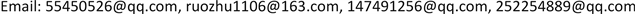Received: Feb. 1st, 2018; accepted: Mar. 6th, 2018; published: Mar. 13th, 2018ABSTRACT

This study combines the hydrodynamic prediction model MIKE21 and the oil spill model OILMAP. Using the hydrodynamic prediction model to provide the accurate tidal current field for oil spill model, we apply oil particle tracing method and stochastic simulation method to predict and evaluate the risk of oil spill in the planned port area. The numerical simulation results show that the current and the wind will directly affect the range and trajectory of the oil film. Once the oil spill accident happens, the area with probability greater than 70% is about 191.5 km2, which is mainly affected by 5 km within two sides of port. Through risk prediction and assessment, we can provide necessary support for the regional emergency forces.

Keywords:oil spill, stochastic simulation, risk prediction, OILMAP1. 引言

2. 数值模拟方法

2.1. 水流控制方程

$\frac{\partial \zeta }{\partial t}+\frac{\partial p}{\partial x}+\frac{\partial q}{\partial y}=\frac{\partial d}{\partial t}$ (1.1)

$\frac{\partial p}{\partial t}+\frac{\partial }{\partial x}\left(\frac{{p}^{2}}{h}\right)+\frac{\partial }{\partial y}\left(\frac{pq}{h}\right)+gh\frac{\partial \zeta }{\partial x}+\frac{gp\sqrt{{p}^{2}+{q}^{2}}}{{C}^{2}\cdot {h}^{2}}-\frac{1}{{\rho }_{w}}\left[\frac{\partial }{\partial x}\left(h{\tau }_{xx}\right)+\frac{\partial }{\partial y}\left(h{\tau }_{xy}\right)\right]-{\Omega }_{q}$ $-fV{V}_{x}+\frac{h}{{\rho }_{w}}\frac{\partial }{\partial x}\left({P}_{\alpha }\right)=0$ (1.2)

$\frac{\partial q}{\partial t}+\frac{\partial }{\partial y}\left(\frac{{q}^{2}}{h}\right)+\frac{\partial }{\partial x}\left(\frac{pq}{h}\right)+gh\frac{\partial \zeta }{\partial y}+\frac{gq\sqrt{{p}^{2}+{q}^{2}}}{{C}^{2}\cdot {h}^{2}}-\frac{1}{{\rho }_{w}}\left[\frac{\partial }{\partial y}\left(h{\tau }_{yy}\right)+\frac{\partial }{\partial x}\left(h{\tau }_{xy}\right)\right]+{\Omega }_{p}$ $-fV{V}_{y}+\frac{h}{{\rho }_{w}}\frac{\partial }{\partial y}\left({P}_{\alpha }\right)=0$ (1.3)

2.2. 溢油模型

Lagrange油粒子运动方程如下：

$X={X}_{0}+\left(U+\alpha {W}_{10}\mathrm{cos}A+r\mathrm{cos}B\right)\Delta t$ (1.4)

$Y={Y}_{0}+\left(U+\alpha {W}_{10}\mathrm{sin}A+r\mathrm{sin}B\right)\Delta t$ (1.5)

OILMAP模型可以对油品的漂移、风化、扩散、溶解、岸线吸附等一系列过程进行模拟，可以预测油膜漂移轨迹和泄漏油品归宿，对其危害程度进行评估，其模拟程序见图1

3. 模型及参数的选取

3.1. 计算域的确定及网格剖分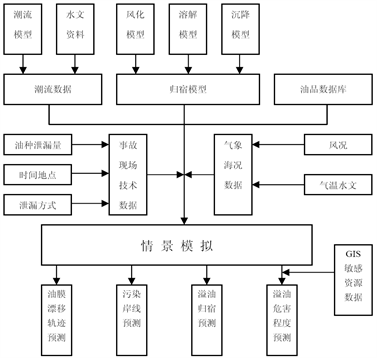Figure 1. Simulation process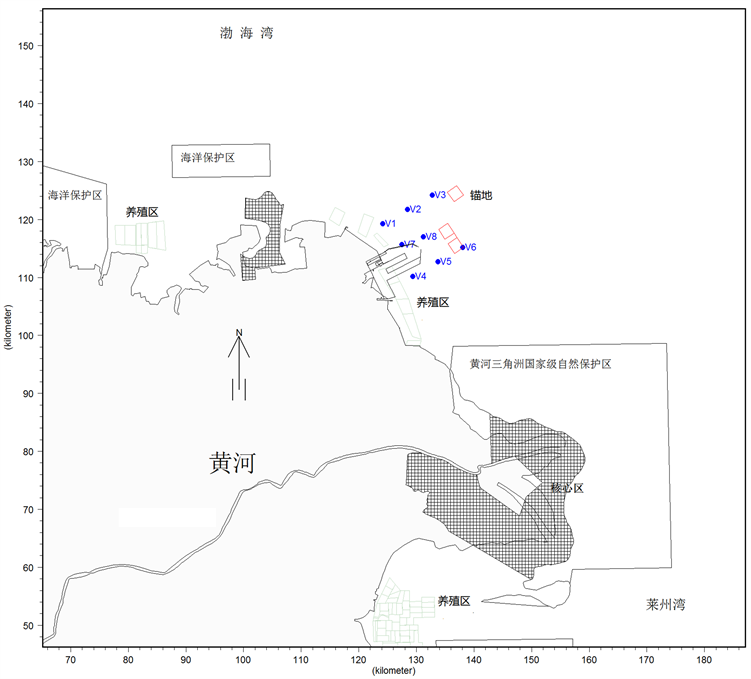Figure 2. Research domain and verification

3.2. 水动力计算参数设置

4. 验证计算及结果分析

4.1. 潮位与流速验证Table 1. Selected coefficient by using mathematical model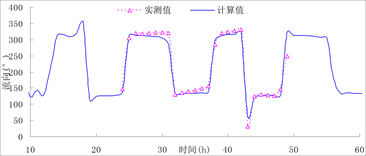Figure 3. V3 station velocity magnitude and directionFigure 4. V6 station velocity magnitude and direction

4.2. 溢油研究区域的流场特征

1) 研究海域位于莱州湾和渤海湾的分界处，黄河三角洲向东北凸入渤海的最端 ，所在海域潮流流速较大；

2) 近岸区域潮流以往复流为主，外海涨潮最大流速可达到0.8 m/s，落潮最大流速可达到0.9 m/s。

3) 港区规划实施前，潮流沿着海岸线方向流动；

4) 港区规划实施后涨、落潮流将受到港区的影响，绕过外侧防波堤进行流动，但在港池内部流速有明显减小趋势；

5) 港区规划会对近岸的潮流流速和流态产生一定的影响，但对港区外海的水动力条件影响较小。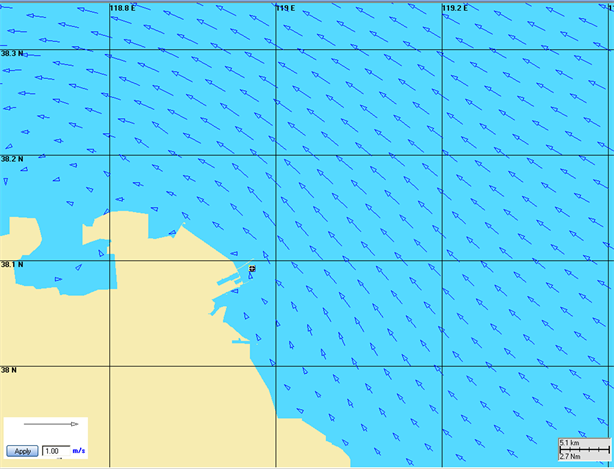Figure 5. Flow filed at flood tide at pre planning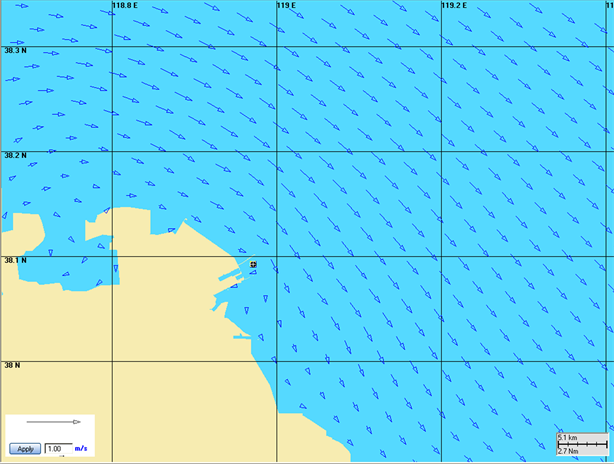Figure 6. Flow filed at ebb tide at pre planning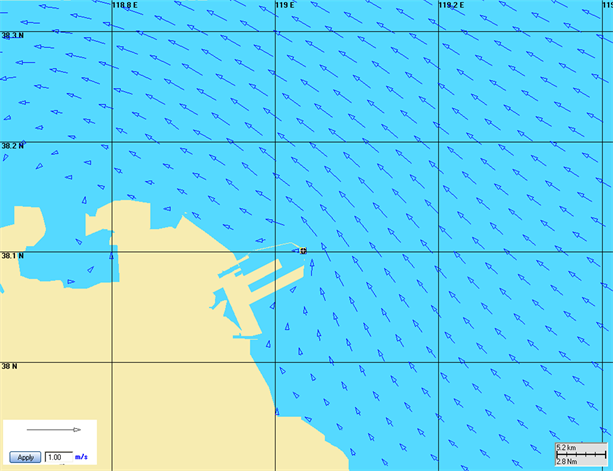Figure 7. Flow filed at flood tide after planningFigure 8. Flow filed at ebb tide after planning

5. 溢油风险影响形势分析

5.1. 污染源项分析

5.2. 典型污染事故后果预测

1) 在全年主导风向为E向、平均风速值6.4 m/s条件下，当溢油事故发生在涨潮时，溢油会随潮流和风的联合作用下将绕过北侧防波堤、很快向正西方向漂移，油膜基本沿着北侧防波堤外侧漂向近岸区域，在溢油24个小时后将抵岸，在整个过程中油膜均不会对北侧现状养殖区和保护区产生直接影响。

2) 当溢油事故发生在落潮时，油膜会在落潮流和风的作用下将向南侧漂移，在19小时后将抵达港区南防波堤处，其后沿着防波堤进行漂移扩散，不会对东营港南北两侧的环境敏感目标产生影响。

3) 在不利风NW风作用下溢油会随潮流和风的联合作用下向东南运动，18小时后进入黄河三角洲海洋保护区(进入时油膜宽约3.6 km)，之后油膜将对该保护区产生持续影响，持续影响时间为49个小时，在此过程中油膜扫海总面积约226.5 km2，漂移距离为62 km。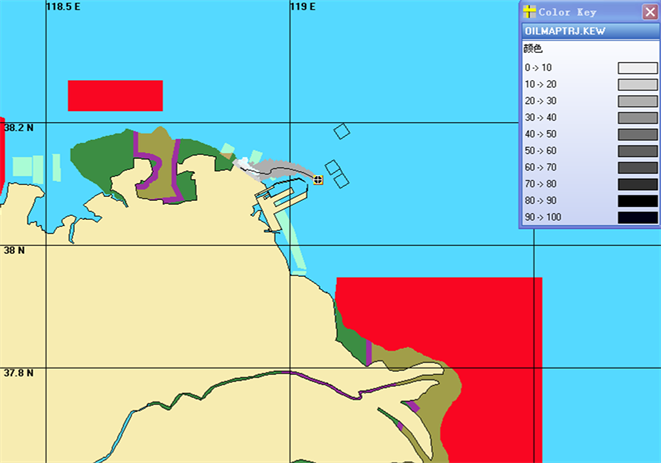Figure 9. Trace and range of oil spill pollution when leading wind E and flood tide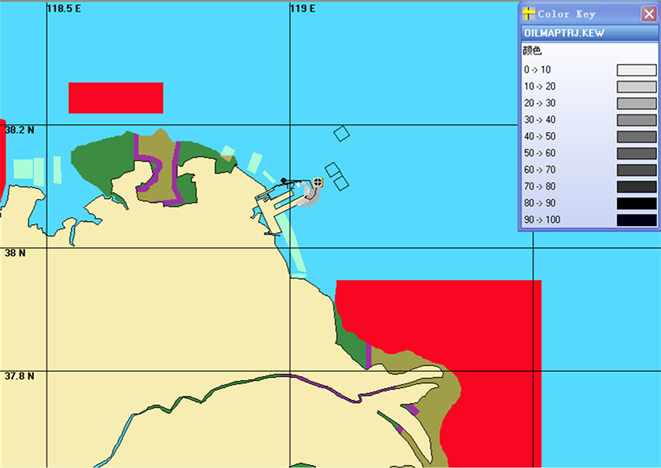Figure 10. Trace and range of oil spill pollution when leading wind E and ebb tide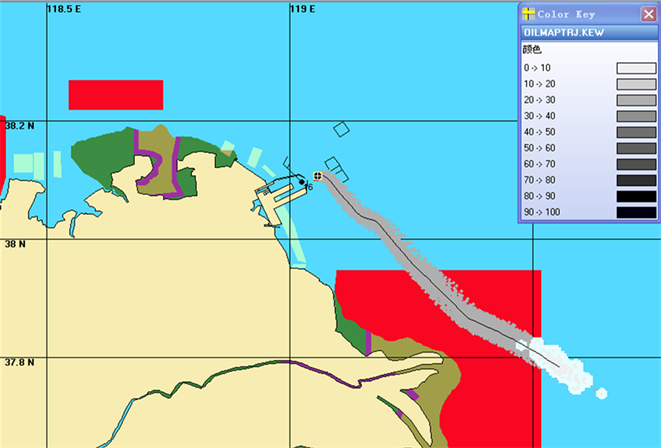Figure 11. Trace and range of oil spill pollution when leading Adverse wind NW and flood tide

5.3. 随机动力条件下的溢油污染概率研究

1) 泄漏位置周围1~30 km，航道两侧5 km范围内受溢油影响的概率超过70%；

2) 在东营港北侧的黄河三角洲国家级自然保护区受溢油影响的概率不超过10%；

3) 在规划港区周边，一旦发生溢油事故，受溢油影响概率大于70%的海域面积约为191.5 km2，主要集中在港区两侧5 km范围内，其他水域受溢油的影响概率较小；

4) 一旦发生溢油事故，将有超过24 km的海岸线有可能受到溢油污染，受影响概率较大的区域主要位于港区北防波堤外侧；

5) 油膜到达港区北侧现状养殖区的最快时间为1~2小时，到达西北侧的黄河三角洲国家级自然保护区最快时间超过4小时。

6. 小结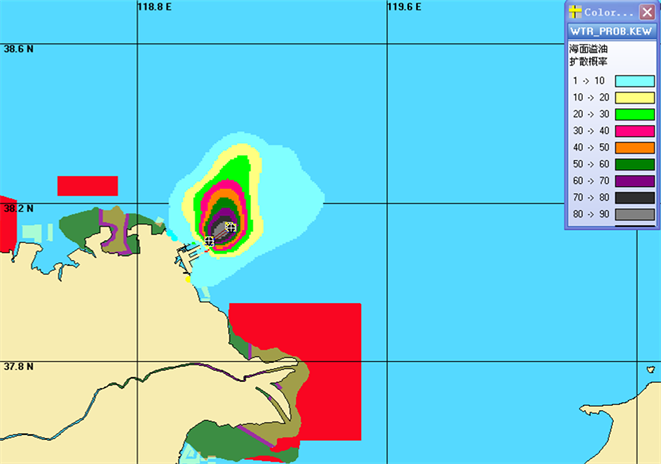Figure 12. Influence probability of oil film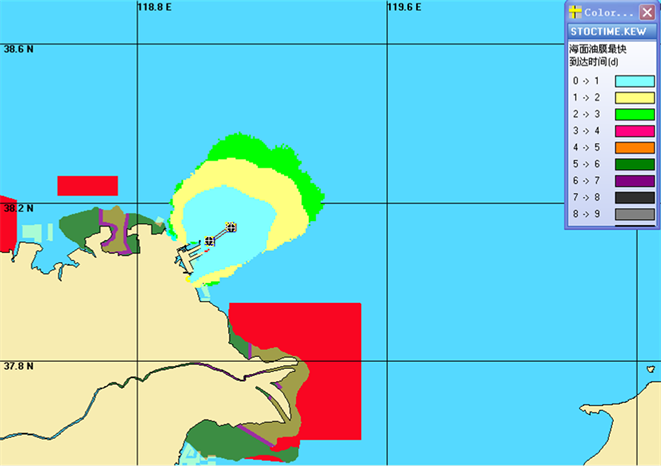Figure 13. The fastest arrival time of oil film

1) 在规划港区实施后，港区规划会对近岸的潮流流速和流态产生一定的影响，但对港区外海的水动力条件影响较小；

2) 在不利风NW风作用下溢油会随潮流和风的联合作用下向东南运动，18小时后进入黄河三角洲海洋保护区；

3) 在规划港区口门处发生海损性事故后，受溢油影响概率大于70%的海域面积约为191.5 km2，其他水域受溢油的影响概率较小；

4) 油膜到达港区北侧现状养殖区的最快时间为1~2小时，到达西北侧的黄河三角洲国家级自然保护区最快时间超过4小时；

The Application of Random Simulation in Predicting the Risk of Oil Spill in Ships[J]. 环境保护前沿, 2018, 08(02): 28-39. http://dx.doi.org/10.12677/AEP.2018.82B003

1. 1. 施欣, 袁群. 长江流域航运水污染影响与治理研究[M]. 上海: 上海交通大学出版社, 2006.

2. 2. 孙建伟, 孙昭晨, 陈轩, 等. NetCDF格式数据的创建及应用[J]. 交通标准化, 2010(15): 31-34.

3. 3. DHI (2009) MIKE21 User Guider. DHI, Copenhagen.

4. 4. 李涛, 李筠, 陈俊峰. 基于OILMAP模型的洋浦港溢油风险研究[J]. 交通节能与环保, 2012, 8(3): 88-89.

5. 5. 范天会. 透空式高桩码头船舶靠离泊数值模拟研究——以东营港扩建工程为例[D]: [硕士学位论文]. 青岛: 中国海洋大学, 2007.

6. 6. JTJ/T233-98 海岸与河口潮流泥沙模拟技术规程[S].

7. 7. 刘菲, 王轲道, 郭传阳, 等. 东营港附近水动力环境特征及其对岸滩地貌的作用[J]. 科学与财富, 2016(8).